PCA

# 白化(Whitening)

Whitening

1. 特征间的相关性较低; (PCA)
2. 所有特征具有相同的方差. ($1\over\sqrt{\lambda_i+\epsilon}$缩放)

PCA已经使得数据间的相关性降低，为使每个输入特征具有单位方差，用$1\over\sqrt{\lambda_i+\epsilon}$作为缩放因子（$\epsilon$用于正则化，防止分母为零，导致数据上溢到无穷，同时具有平滑即低通滤波作用），缩放每个特征$x_{rot,i}$，即PCA白化后的数据$x_{PCAwhite}$满足：

$x_{PCAwhite,i}={x_{rot,i}\over\sqrt{\lambda_i+\epsilon}}, \space i代表成分或维度$

PCA白化后的数据已经具有单位协方差，ZCA白化是在PCA白化的基础上，左乘一个任意正交矩阵$R$$RR^{T}=R^{T}R=I$，也可以是旋转或反射矩阵），那么处理后的数据仍然具有单位协方差，在ZCA白化中，取PCA投影矩阵$R=U$，则：

$x_{ZCAwhite}=Ux_{PCAwhite}$

# PCA/Whitening 计算步骤

Implementing
PCA/Whitening

1. 使数据均值为零;
2. 计算协方差矩阵;
3. 计算协方差矩阵的特征值和特征向量;
4. 使用特征向量组成的PCA变换矩阵$U$（旋转矩阵，按特征值大小降序且按列排）旋转数据，得到PCA变换后的数据$x_{rot}$，取前$k$个特征向量组成旋转矩阵旋转数据，得到PCA降维后的数据（假设输入$x\in R^n, \space k<n$）;
5. 使用$1\over\sqrt{\lambda_i+\epsilon}$对PCA旋转后的数据缩放，使其具有相同单位协方差，得到PCA白化后的数据$x_{PCAwhite}$;
6. 对PCA白化后的数据，左乘PCA变换矩阵得到ZCA白化后的数据$x_{ZCAwhite}$.

# 二维数据PCA实验

Exercise:PCA_in_2D

MATLAB实现：

• 使数据均值为零：（提供的二维数据以具有相同均值0，此步省去）

avg = mean(x, 1);
x = x - repmat(x, 1);

• 计算协方差矩阵：

sigma = cov(x', 1); % MATLAB function, '1' normalize with n
sigma = x*x'/size(x, 2); % or you can use this code


原始数据协方差：

sigma =
0.0883    0.0733
0.0733    0.0890

• 计算协方差矩阵的特征值和特征向量：

[U, S, ~] = svd(sigma);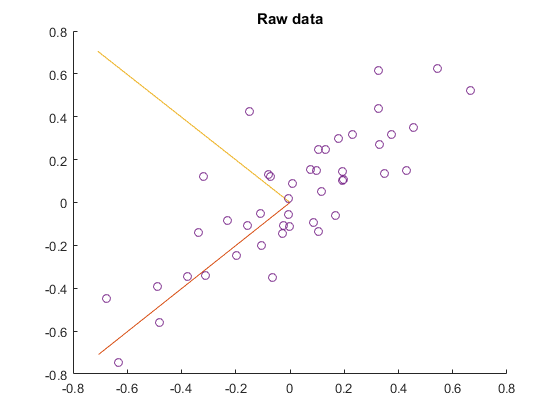U =
-0.7055   -0.7087
-0.7087    0.7055
S =
0.1620         0
0    0.0154

• PCA变换旋转数据$x$得到$x_{rot}$

xRot = U'*x;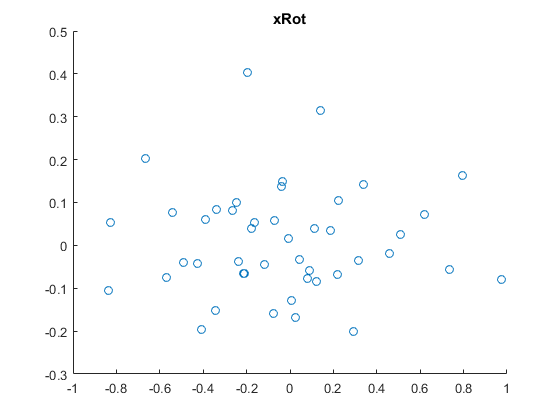PCA旋转后的数据

• PCA降维数据:
xRot = U(:,1:k)'*x; % projecting to 1 dimension
xHat = U(:,1:k)*xRot; % projecting the xRot back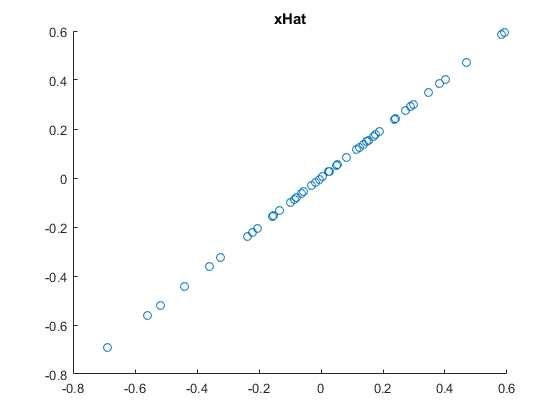PCA降维 - 旋转后映射回的数据

• PCA白化：
xPCAWhite = diag(1./sqrt(diag(S)+epsilon))*U'*x; % xPCAWhite_i = xRot_i/sqrt(lambda_i)

sigmaPCAWhite = cov(xPCAWhite', 1); % computes the covariance of PCAWhite data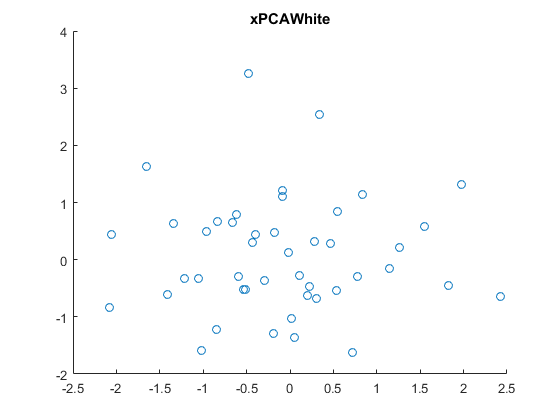PCA降维 - PCA白化后的数据

sigmaPCAWhite =
0.9921    0.0066
0.0066    0.9937

• ZCA白化：
xZCAWhite = U*diag(1./sqrt(diag(S)+epsilon))*U'*x; % xZCAWhite_i = U*xPCAWhite

sigmaZCAWhite = cov(xZCAWhite', 1); % computes the covariance of ZCAWhite data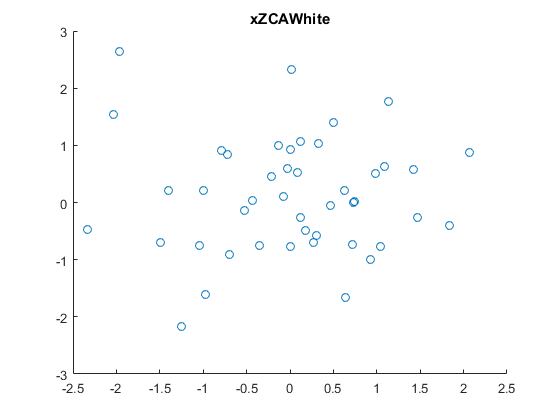ZCA降维 - ZCA白化后的数据

sigmaZCAWhite =
0.9996   -0.0008
-0.0008    0.9863


# PCA与白化图像数据实验

Exercise:PCA and
Whitening

## PCA实验

$Cov(X,Y)=E[(X-E[X])(Y-E[Y])] ,$

So, we won’t use variance normalization. The only normalization we
need to perform then is mean normalization, to ensure that the
features have a mean around zero
. Depending on the application, very
often we are not interested in how bright the overall input image is.
For example, in object recognition tasks, the overall brightness of
the image doesn’t affect what objects there are in the image. More
formally, we are not interested in the mean intensity value of an
image patch; thus, we can subtract out this value, as a form of mean
normalization
.

## PCA白化实验

Exercise: PCA
Whitening

MNIST DATABASE

• 零均值化数据

% avg = mean(x, 1);
% x = x - repmat(avg, size(x,1), 1);
x = bsxfun(@minus, x, mean(x,1)); % or this code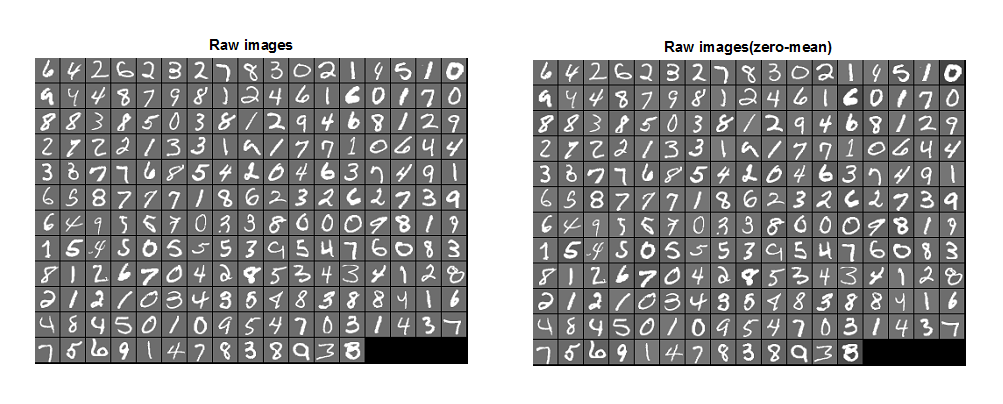• 旋转数据：
sigma = x*x'/m; % n-by-n
[u, s, ~] = svd(sigma);
xRot = u'*x;% n-by-m

• 验证PCA实现的正确性，即旋转数据的协方差仅对角元素非零：
covar = xRot*xRot'/m; % the mean of xRot is 0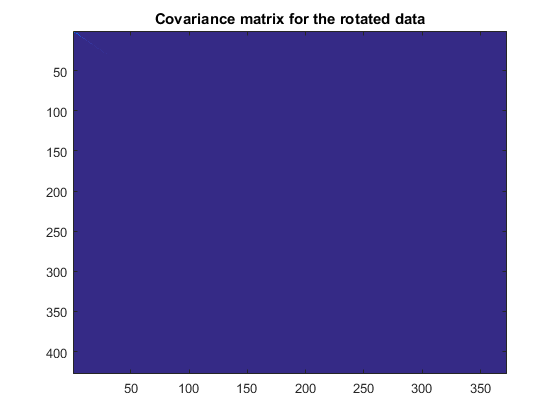• 寻找保留主成分数目：

lambda = diag(s);
lambda_sum = sum(lambda);
lambda_sumk = 0;
perc = 0;
k = 0;
while perc < 0.99
k = k + 1;
lambda_sumk = lambda_sumk + lambda(k);
perc = lambda_sumk / lambda_sum;
end

• 维数约简与重构：
xDimk = u(:,1:k)'*x;
xHat = u(:,1:k)*xDimk;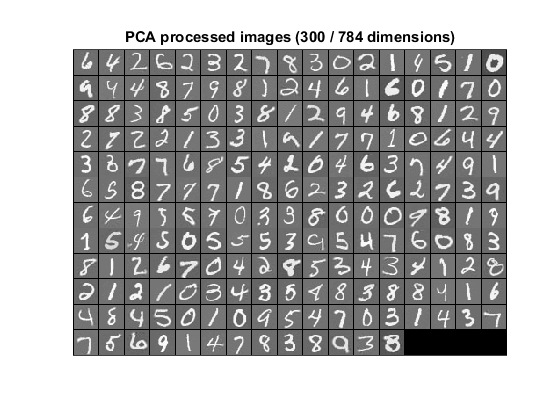PCA维数约简后重构的图像 (采用每个图像块的均值零均值化图像,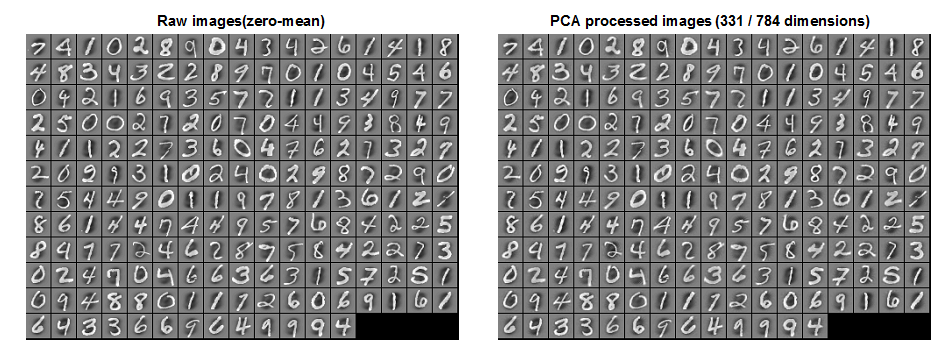PCA维数约简后重构的图像 (采用所有图像块的均值零均值化图像,

• PCA白化及检验：

epsilon = 1e-1;
xPCAWhite = diag(1./sqrt(diag(s)+epsilon))*u'*x;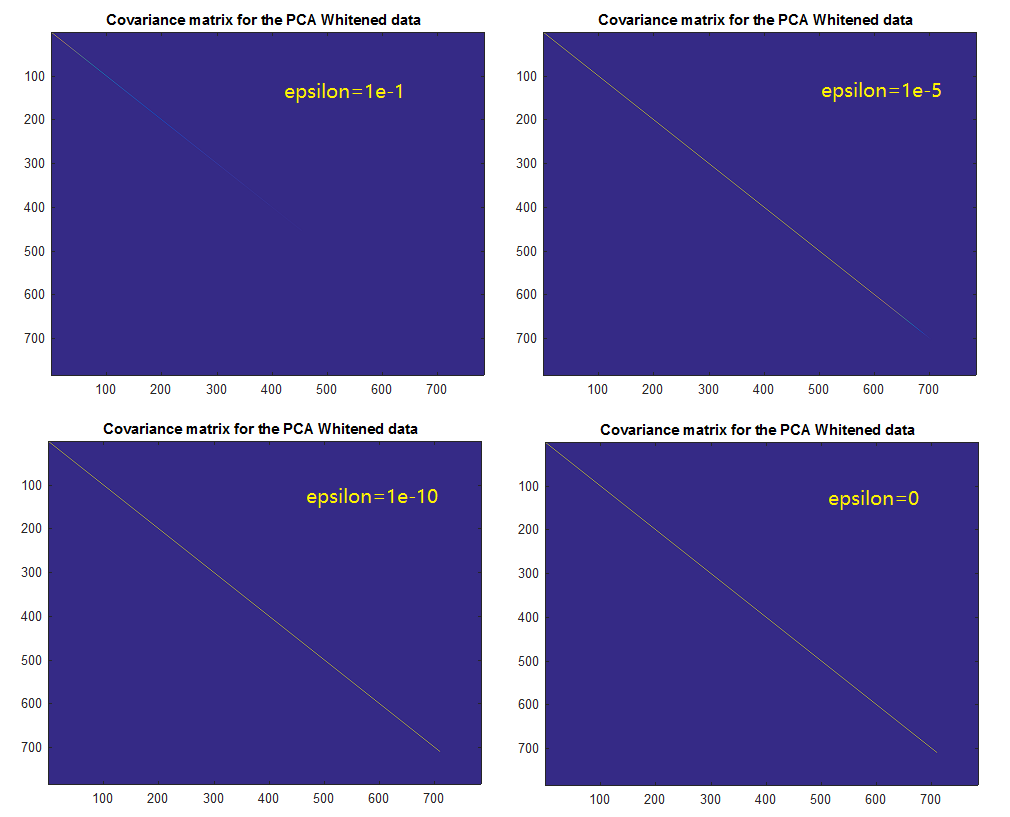• ZCA白化：
xZCAWhite = u*diag(1./sqrt(diag(s)+epsilon))*u'*x;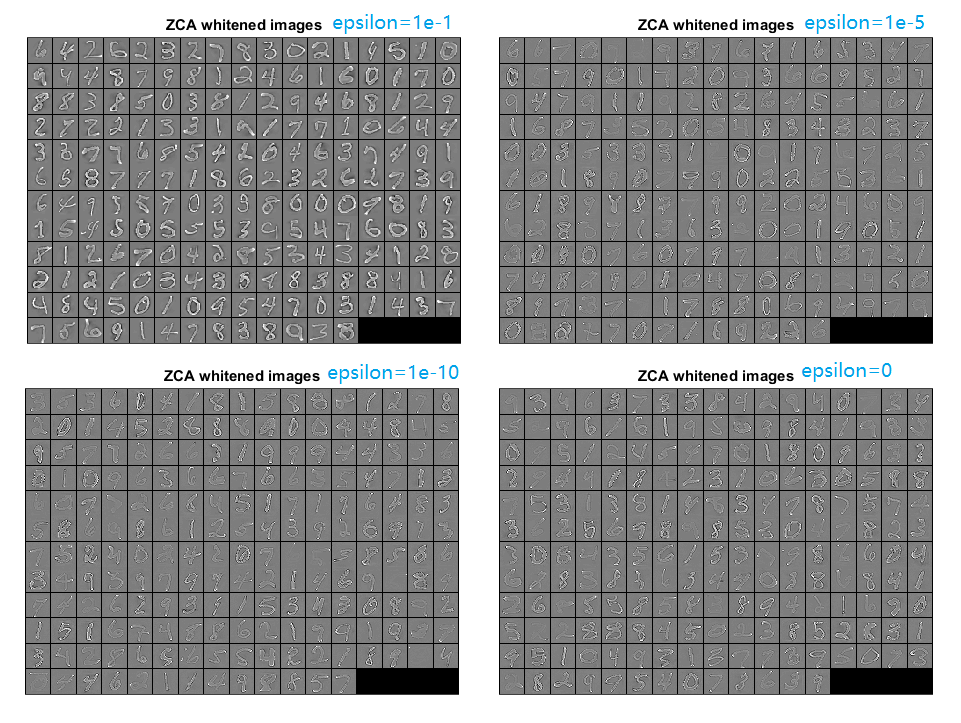ZCA维数约简后重构的图像

©️2019 CSDN 皮肤主题: 大白 设计师: CSDN官方博客